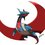# READ ME

this is part of the set @the Radicals  let there be a infinite Radical $\sqrt{17+2\sqrt{88+24\sqrt{17+2\sqrt{88+24\sqrt{\dots\dots }}}}}$ it can be written as $\sqrt{3^2+2^2+2^2+2\sqrt{(3\times2)^2+(2\times 2)^2 +(3\times 2)^2 +2(3\times 2\times 2)\sqrt{\dots\dots }}}$ then we can say that $\sqrt{17+2\sqrt{88+24\sqrt{17+2\sqrt{88+24\sqrt{\dots\dots }}}}}=7$ how??? lets see let $F(a,b,c)=a+b+c$ $F(a,b,c)=\sqrt{a^2 +b^2+c^2 +2(ab+bc+ca)}$ $F(a,b,c)=\sqrt{a^2 +b^2 +c^2 +2\sqrt{(ab)^2+(bc)^2+(ca)^2+2(a^2bc+ab^2c+abc^2)}}$ $F(a,b,c)=\sqrt{a^2 +b^2 +c^2 +2\sqrt{(ab)^2+(bc)^2+(ca)^2+2abc(a+b+c)}}$ now we can expand $$a+b+c$$ like before and make it an infinite radical so we can say that$\sqrt{a^2 +b^2 +c^2 +2\sqrt{(ab)^2+(bc)^2+(ca)^2+2abc\sqrt{a^2 +b^2 +c^2 +2\sqrt{(ab)^2+(bc)^2+(ca)^2+2abc\sqrt{\dots\dots}}}}} =a+b+c$Note by Aareyan Manzoor
6 years, 3 months ago

This discussion board is a place to discuss our Daily Challenges and the math and science related to those challenges. Explanations are more than just a solution — they should explain the steps and thinking strategies that you used to obtain the solution. Comments should further the discussion of math and science.

When posting on Brilliant:

• Use the emojis to react to an explanation, whether you're congratulating a job well done , or just really confused .
• Ask specific questions about the challenge or the steps in somebody's explanation. Well-posed questions can add a lot to the discussion, but posting "I don't understand!" doesn't help anyone.
• Try to contribute something new to the discussion, whether it is an extension, generalization or other idea related to the challenge.
• Stay on topic — we're all here to learn more about math and science, not to hear about your favorite get-rich-quick scheme or current world events.

MarkdownAppears as
*italics* or _italics_ italics
**bold** or __bold__ bold
- bulleted- list
• bulleted
• list
1. numbered2. list
1. numbered
2. list
Note: you must add a full line of space before and after lists for them to show up correctly
paragraph 1paragraph 2

paragraph 1

paragraph 2

[example link](https://brilliant.org)example link
> This is a quote
This is a quote
    # I indented these lines
# 4 spaces, and now they show
# up as a code block.

print "hello world"
# I indented these lines
# 4 spaces, and now they show
# up as a code block.

print "hello world"
MathAppears as
Remember to wrap math in $$ ... $$ or $ ... $ to ensure proper formatting.
2 \times 3 $2 \times 3$
2^{34} $2^{34}$
a_{i-1} $a_{i-1}$
\frac{2}{3} $\frac{2}{3}$
\sqrt{2} $\sqrt{2}$
\sum_{i=1}^3 $\sum_{i=1}^3$
\sin \theta $\sin \theta$
\boxed{123} $\boxed{123}$

## Comments

Sort by:

Top Newest

$x+a=\sqrt{(x+a)^3}\\x+a=\sqrt{x^3+3ax^2+3a^2x+a^3}\\x+a=\sqrt{x^3+2ax^2+ax^2+3a^2x+a^3}\\x+a=\sqrt{ax^2+3a^2x+a^3+x^3+2ax^2}\\x+a=\sqrt{a(x^2+3ax+a^2)+x^2(x+2a)}$ In the same way get $x+2a=\sqrt{2a(x^2+3(2a)x+(2a)^2)+x^2(x+4a)}$ and $x+4a=\sqrt{4a(x^2+3(4a)x+(4a)^2)+x^2(x+8a)}$ and the expressions for $x+8a,x+16a\;etc$.Replacing all of these in the original expression we get: $\color{#D61F06}{x+a=\sqrt{a(x^2+3ax+a^2)+x^2\sqrt{2a(x^2+3(2a)x+(2a)^2)+x^2\sqrt{4a(x^2+3(4a)x+(4a)^2)+x^2\sqrt{\dotsm\;to\; \infty}}}}}$ $\LARGE{\color{#20A900}{V}\color{#3D99F6}{O}\color{#624F41}{I}\color{#69047E}{L}\color{#D61F06}{A}\color{#333333}{!!!}}$

- 6 years, 3 months ago

Log in to reply

Please comment @Aareyan Manzoor

- 6 years, 3 months ago

Log in to reply

ok, i find these seriously fascinating, the one by you is 1 of many. it is really good as well, let me show you another $a+b =\sqrt{a^3 +b^3 +3ab(a+b)}$ $a+b=\sqrt{a^3 +b^3 +3ab\sqrt{a^3 +b^3 +3ab\sqrt{a^3 +b^3 +3ab\sqrt{\dots\dots}}}}$

- 6 years, 3 months ago

Log in to reply

Nice.I was just fixated on nested radicals when I saw the one by Ramanujan.I find them seriously beautiful.

- 6 years, 3 months ago

Log in to reply

How about this one: $x=\sqrt{1+(x-1)(x+1)}\\=\sqrt{1+(x-1)\sqrt{1+x(x+2)}}\\=\sqrt{1+(x-1)\sqrt{1+x\sqrt{1+(x+1)(x+3)}}}\\=\sqrt{1+(x-1)\sqrt{1+x\sqrt{1+(x+1)\sqrt{1+(x+2)(x+4)}}}}$ Continuing this indefinitely we get: $x=\sqrt{1+(x-1)\sqrt{1+x\sqrt{1+(x+1)\sqrt{1+(x+2)\sqrt{\dotsm}}}}}$

- 6 years, 3 months ago

Log in to reply

already taken by ramnujan $\sqrt{ax+(a+n)^2+x\sqrt{ax+(a+n)^2+(x+n)\sqrt{\dots\dots}}}=x+n+a$

- 6 years, 3 months ago

Log in to reply

Yeah,realized that after posting the comment.

- 6 years, 3 months ago

Log in to reply

nice one

- 6 years, 3 months ago

Log in to reply

Please note that just because something continues ad infinitum, you cannot add the $\inf$ symbol there. That'd mean you're literally having the hyperreal number infinity in the expresssion

- 6 years, 3 months ago

Log in to reply

thanks, by the way, what is a hyperreal number,sir?

- 6 years, 3 months ago

Log in to reply

Hyperreal Numbers (denoted $*\mathbb{R}$ is an extension of Real Numbers to accomodate infinite and infinitesimal numbers. It's an interesting idea, you could look it up.

- 6 years, 3 months ago

Log in to reply

KAGE BUNSHIN NO JUTSU

- 5 years, 3 months ago

Log in to reply

×

Problem Loading...

Note Loading...

Set Loading...